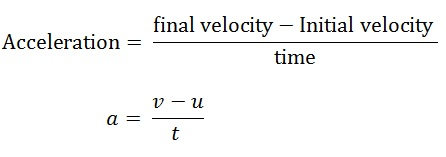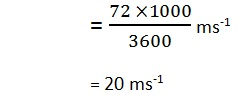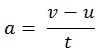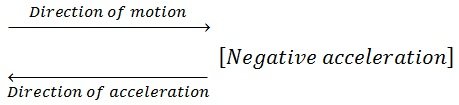Study Materials: CBSE Notes

Our CBSE Notes for Notes 8. Motion - Accelaration | Class 9 Science - Toppers Study is the best material for English Medium students cbse board and other state boards students.

Notes ⇒ Class 9th ⇒ Science ⇒ 8. Motion

# Notes 8. Motion - Accelaration | Class 9 Science - Toppers Study

Topper Study classes prepares CBSE Notes on practical base problems and comes out with the best result that helps the students and teachers as well as tutors and so many ecademic coaching classes that they need in practical life. Our CBSE Notes for Notes 8. Motion - Accelaration | Class 9 Science - Toppers Study is the best material for English Medium students cbse board and other state boards students.

## Notes 8. Motion - Accelaration | Class 9 Science - Toppers Study

CBSE board students who preparing for class 9 ncert solutions maths and Science solved exercise chapter 8. Motion available and this helps in upcoming exams 2023-2024.

### You can Find Science solution Class 9 Chapter 8. Motion

• All Chapter review quick revision notes for chapter 8. Motion Class 9
• NCERT Solutions And Textual questions Answers Class 9 Science
• Extra NCERT Book questions Answers Class 9 Science
• Importatnt key points with additional Assignment and questions bank solved.

Chapter 8 Science class 9

### Accelaration class 9 Science Chapter 8. Motion

• Notes 8. Motion - Accelaration | Class 9 Science - Toppers Study
• Class 9 Ncert Solutions
• Solution Chapter 8. Motion Class 9
• Solutions Class 9
• Chapter 8. Motion Accelaration Class 9

## Notes 8. Motion - Accelaration | Class 9 Science - Toppers Study

Acceleration :

Acceleration is changing in the velocity of an object per unit time is called acceleration.

In other words:

The rate of changing in the velocity is known as acceleration.

• The SI unit ofacceleration is m s-2.
• It is denoted by a.
• Acceleration is a vector quantity.

Accelerated motion: If the velocity of an object changes from an initial value u to the final value v in time t, the acceleration is a, and this kind of motion is known as accelerated motion.Example: A bus starts from rest and attains a speed of 72 km h-1 in 5 minutes while moving in uniform motion. Find the acceleration of the bus.

Solution:

Time (t) = 5 minites = 5 × 60 = 300 seconds

Initial velocity u = 0    [∵ bus was in rest]

Final velocity v = 72 km h-1= (20 - 0)/300

= 0.066 ms-2

Acceleration of object = 0.066 ms-2

Type of accelerations are based on direction of acceleration:

These are:

(I) Positive acceleration

(II) Negative acceleration

(I) Positive acceleration : If the velocity of an object increases with time in the direction of motion of the object, this is known as positive acceleration.

The direction of acceleration is same as the direction of motion.Main points:

• The direction of acceleration is same as the direction of motion.
• When the velocity increases with time in the same direction.
• When an object starts from rest.
• The final velocity is greater than initial velocity i.e (v > u).

(II) Negative acceleration : If the velocity of an object decreases with time in an opposite direction of motion of the object, this is known as negative acceleration.

Retardation: Negative accelaration is known as retardation.Main points:

• The direction of acceleration is opposite to the direction of motion.
• Negative accelaraion produces when the velocity decreases with time.
• When an object is going to rest.
• The final velocity is smaller than initial velocity i.e (v < u).

Uniform accelaration: When the velocity of an object changes by an equal amount in equal intervals of time such a accelaration of the object is known as uniform accelaration.

Uniformly accelarated motion: The motion of an object having uniform accelaration is known as uniformly accelarated motion.

Examples:

(i) motion of a rolling ball on smooth surface.

(ii) The motion of a freely falling body.

(iii) Motion of the earth round the sun.

(iv) A high speed lift starts from multi-storied building.

(v) The motion of the moon round the Earth.

Non-uniform accelaration: When the velocity of an object changes by an unequal amount in equal intervals of time such a accelaration of the object is known as non-uniform accelaration.

Non-uniformly accelarated motion: The motion of an object having different accelaration is known as non-uniformly accelarated motion.

Examples:

(i) A racing car.

(ii) The motion of vehicles moving at differents speeds.

(iii) The ball is thrown upward at top it get retarded.

(iv) The motion of a bus passing through rough road.

Important Study materials for classes 06, 07, 08,09,10, 11 and 12. Like CBSE Notes, Notes for Science, Notes for maths, Notes for Social Science, Notes for Accountancy, Notes for Economics, Notes for political Science, Noes for History, Notes For Bussiness Study, Physical Educations, Sample Papers, Test Papers, Mock Test Papers, Support Materials and Books.Mathematics Class - 11th

NCERT Maths book for CBSE Students.

books

## Study Materials List:

##### Notes ⇒ Class 9th ⇒ Science
1. Matter in Our Surroundings
2. Is Matter around us Pure
3. Atoms and Molecules
4. Structure of The Atom
5. The Fundamental Unit of Life
6. Tissues
7. Diversity in Living Organisms
8. Motion
9. Force and Laws of Motion
10. Gravitation
11. Work and Energy
12. Sound
13. Why Do We Fall ill
14. Natural Resources
15. Improvement in Food Resources

New Books